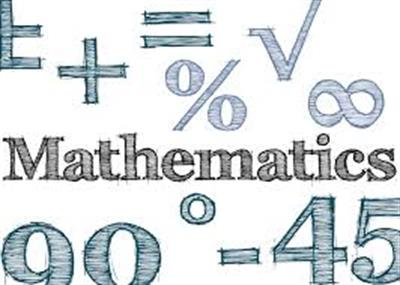Search - School / College / Institute

# A FEW INTERESTING FACTS ABOUT MATHEMATICSGoogle got its name from the word ‘googol’, which means that the number one is followed by 100 zeros. It reflects the possibility of infinite searches over the net.

Maths gets crazy with its functions. For example, when 111,111,111 is multiplied by 111,111,111, the resultant number is 12,345,678,987,654,321.

Americans call it ‘math’ and the British ‘maths’. The former believe that it is a singular noun, but the latter reason it as a plural terms as it ends with the letter ‘s’. When the number 1 gets divided by 998,001, the resultant number is a complete sequence from 000 to 999 in order.

You can calculate a pizza’s volume with the formula- Pi x r2 x h. If a pizza’s height is ‘a’ and radius ‘z’, then its volume is Pi x z x z x a. 9 is believed to be a magical number. If you multiply any number with 9, and add up the resulting number’s digits, the sum would be 9.

The Roman numerals do not represent zero, which is one of the most important numbers. Billion, trillion, quadrillion, quintillion, sextillion, septillion, octillion, nonillion, decillion, and undecillion – these come after a million.

Content: indiatoday.intoday.in### Future Bright Program

Enhance Your Skills With Our ExpertsInteractive School Platform